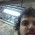## Tuesday, August 6, 2019

### What is the mass of a molecular vibration?

This is a basic question that I have been puzzling about. I welcome solutions.

Consider a diatomic molecule containing atoms with mass m1 and m2. It has a stretch vibration that can be described by a harmonic oscillator with a reduced mass mu given by
Now consider a polyatomic molecule containing N atoms.
It will have 3N-6 normal modes of vibration.
[The 6 is due to the fact that there are 6 zero-frequency modes: 3 rigid translations and 3 rotations of the whole molecule].
In the harmonic limit, the normal mode problem is solved below.
[I follow the classic text Wilson et al., Molecular Vibrations].
The problem is also solved in matrix form in Chapter 6 of Goldstein, Classical Mechanics].

One now has a collection of non-interacting harmonic oscillators. All have mass = 1. This is because the normal mode co-ordinates have units of length * sqrt(mass).

The quantum chemistry package Gaussian does more. It calculates a reduced mass mu_i for each normal mode i using the formula below.
This is discussed in these notes on the Gaussian web site. From mu_i and the normal mode frequency_i it then calculates the spring constant for each normal mode.

I have searched endlessly, and tried myself, but have not been able to answer the following basic questions:

1. How do you derive this expression for the reduced mass?
2. Is this reduced mass physical, i.e. a measurable quantity?

Similar issues must also arise with phonons in crystals.

Any recommendations?

#### 3 comments:

1.Ehm... It's elementary physics. If you have the kinetic energy of two bodys that have masses m1 & M2, and velocities v1 & V2, T=½m1•v1²+½m2•v2². The centre of mass has velocity (m1•v1+m2•v2)/(m1+m2). If you want a mass asociated with v1-v2, preserving the same kinetic energy, where the other mass is m1+M2 with velocity of centre of mass, the mass you get is the reduced mass. Ir works the same for non-relativistic Quantum mechanics (when you change vi for -i\frac{\hbar\nabla}{mi}).

2.Once you have a total speed V, you can define a reduced mass μ so that the kinetic energy is T = ½μV². I think the crux is how to add up the particle speeds.

In the two particle case, say that one particle has mass m and is going left with speed v₁=Mu, and the other has mass M and is going right with speed v₂=mu. (I suggest readers get a pen and draw that.) The centre of mass is stationary.

Then T = ½m·(Mu)² + ½M·(mu)² = ½ mM/(M+m)·(mu+Mu)² = ½μ(v₁+v₂)². In the simplest case, you just add up the particle speeds.

So this might be a start. Pick a time when the kinetic energy of the classical vibrational mode is a maximum. The particles have masses m_i and speeds v_i. Choose μ such that ∑_i m_i·v_i² = μ·(∑_i v_i)².

3.For a phonon with dispersion relation ω(k), there's a kind of group dispersion. Define the mass μ of the mode with wave number k such that ω(k+k') = ω(k) + v_g·k' + (ℏ/2μ)·k'² + O(k'³). I don't know how that relates to the classical version.